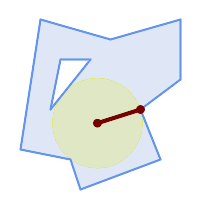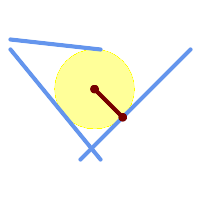## Name

ST_MaximumInscribedCircle — Computes the largest circle contained within a geometry.

## Synopsis

`(geometry, geometry, double precision) ST_MaximumInscribedCircle(`geometry geom`)`;

## Description

Finds the largest circle that is contained within a (multi)polygon, or which does not overlap any lines and points. Returns a record with fields:

• `center` - center point of the circle

• `nearest` - a point on the geometry nearest to the center

• `radius` - radius of the circle

For polygonal inputs, the circle is inscribed within the boundary rings, using the internal rings as boundaries. For linear and point inputs, the circle is inscribed within the convex hull of the input, using the input lines and points as further boundaries.

Availability: 3.1.0.

Requires GEOS >= 3.9.0.

## ExamplesMaximum inscribed circle of a polygon. Center, nearest point, and radius are returned.

```SELECT radius, ST_AsText(center) AS center, ST_AsText(nearest) AS nearest
FROM ST_MaximumInscribedCircle(
'POLYGON ((40 180, 110 160, 180 180, 180 120, 140 90, 160 40, 80 10, 70 40, 20 50, 40 180),
(60 140, 50 90, 90 140, 60 140))');